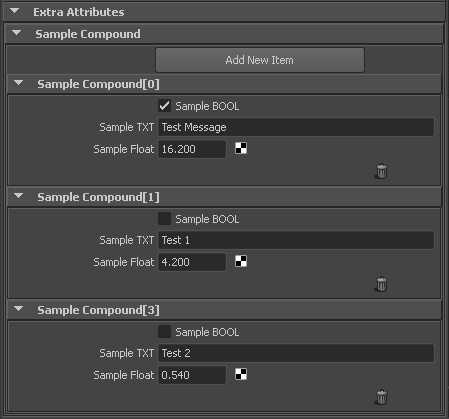#### OpenMaya.MTransformationMatrix Rotation matrix from 3 vectorsSmall practical example with Maya API python functionalities
Generate Rotation matrix using 3 vectors (normal, tangent, up)

#### Create png file using OpenMaya.MImage()

```import maya.OpenMaya as OpenMaya

def main():
m_color = ( 0.0, 1.0, 0.0, 1.0 )
m_color = float4Torgba(m_color)
generateTexture("c:/tmp","color01",m_color)

def generateTexture(m_path,m_fileName,m_color):
try:
m_util = OpenMaya.MScriptUtil
#-------------
m_height = 16
m_width = 16
m_depth = 4
#-------------
m_image = OpenMaya.MImage()
m_image.create(m_height, m_width, m_depth )
m_pixels = m_image.pixels()
#-------------
m_arrayLen = m_width * m_height * m_depth
for i in range(0, m_arrayLen, m_depth):
m_util.setUcharArray(m_pixels, i+0, m_color)
m_util.setUcharArray(m_pixels, i+1, m_color)
m_util.setUcharArray(m_pixels, i+2, m_color)
m_util.setUcharArray(m_pixels, i+3, 0)
m_image.setPixels(m_pixels, m_height, m_width)
m_image.writeToFile( "{}/{}.png".format(m_path,m_fileName), '.png' )
except:
OpenMaya.MGlobal.displayWarning("Can't save file to {}/{}.png".format(m_path,m_fileName))
return False
else:
return True

def float4Torgba((m_r,m_g,m_b,m_a)):
m_red   = int(m_r * 255.0)
m_green = int(m_g * 255.0)
m_blue  = int(m_b * 255.0)
m_alpha = int(m_a * 255.0)
return (m_red, m_green, m_blue, m_alpha)

main()
```

#### Attach Polygons Tool for Maya like in 3ds Max

The same like in 3dsmax. Attach polygons via Maya Python Api and Maya cmds to existing mesh.
Not combine, no cheating. Also with skinning support.
Limitation : very slow.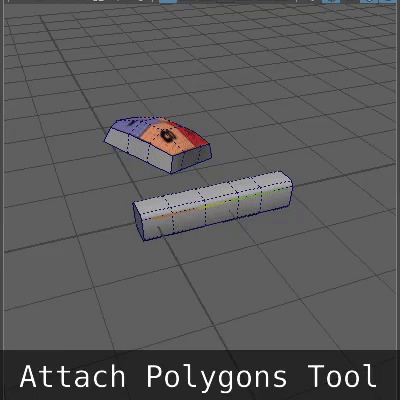Just unzip the script into C:/maya_scripts/modeling and make a Python shelf button in Maya with this code:

```import sys
import maya.cmds as cmds
path = 'C:/maya_scripts/modeling'
if path not in sys.path:
sys.path.append(path)
import attachObjects; attachObjects.main();
```

#### Generate random uv’s Maya Python API

Quick example working with OpenMaya.MFnMesh setUV and assignUV.
Limitation : works only on objects with no construction history.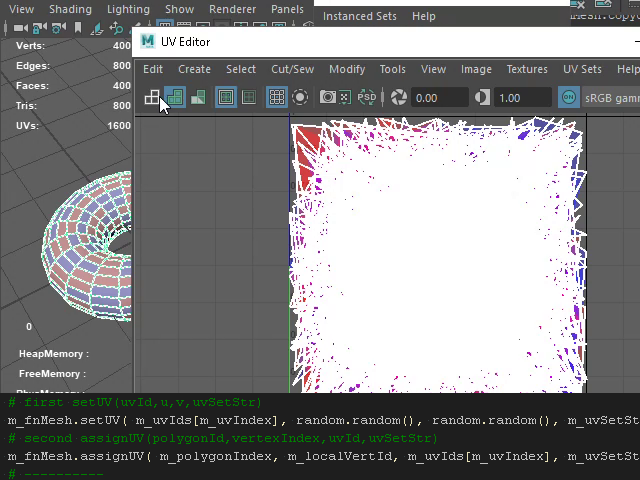#### Convert Vertex Normals to Soft/Hard Edges using Maya Python API

Usage:

```
import vtxNormalsToSoftHardEdges; vtxNormalsToSoftHardEdges.convertNormals();
```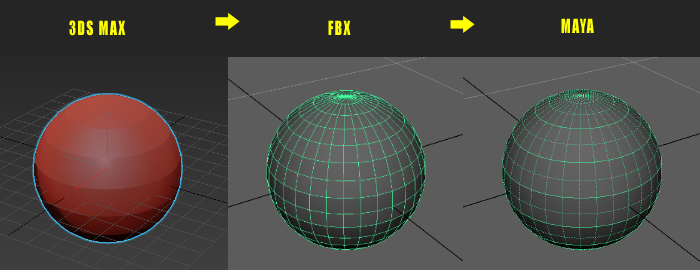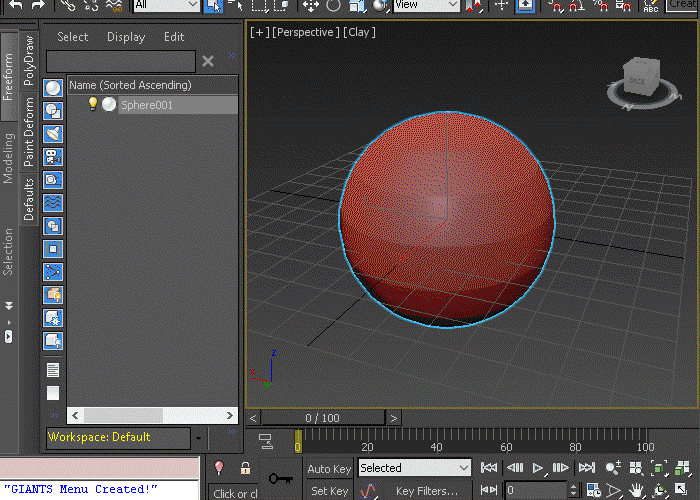#### get MDagPath() from MObject()

Quick example

```
import maya.OpenMaya as OpenMaya

m_obj       = OpenMaya.MObject() # should get from any code above
m_dagPath   = OpenMaya.MDagPath()
if m_obj.hasFn( OpenMaya.MFn.kDagNode ):
OpenMaya.MDagPath.getAPathTo( m_obj, m_dagPath )
print( m_dagPath.fullPathName() )
```

#### Traversing whole scene file Maya Python API

Quick example

```
import maya.OpenMaya as OpenMaya

m_iterator = OpenMaya.MItDag( OpenMaya.MItDag.kDepthFirst )
while not m_iterator.isDone():
m_obj   = m_iterator.currentItem()
m_objFn = OpenMaya.MFnDagNode( m_obj )
#if ( OpenMaya.MFn.kMesh == m_obj.apiType() ):
print( m_objFn.fullPathName() )
m_iterator.next()
```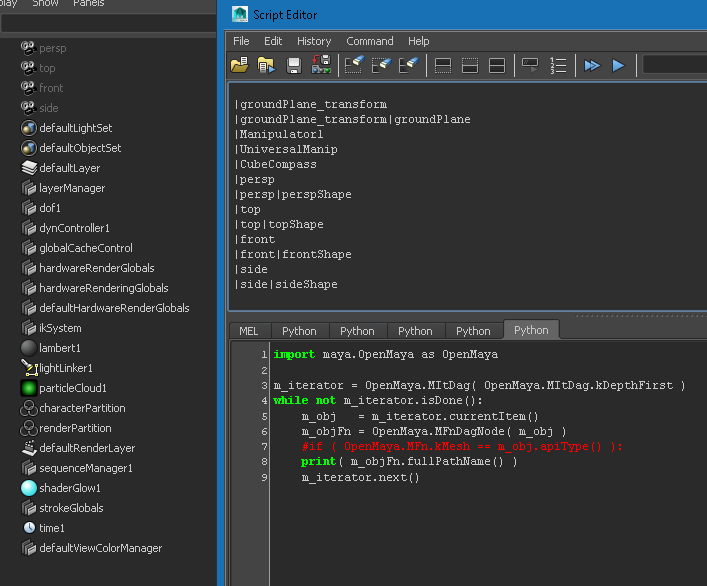#### Get all extra attributes Maya Python API

Select the node and run:

```
m_list = getAllExtraAttributes()
print( m_list )
```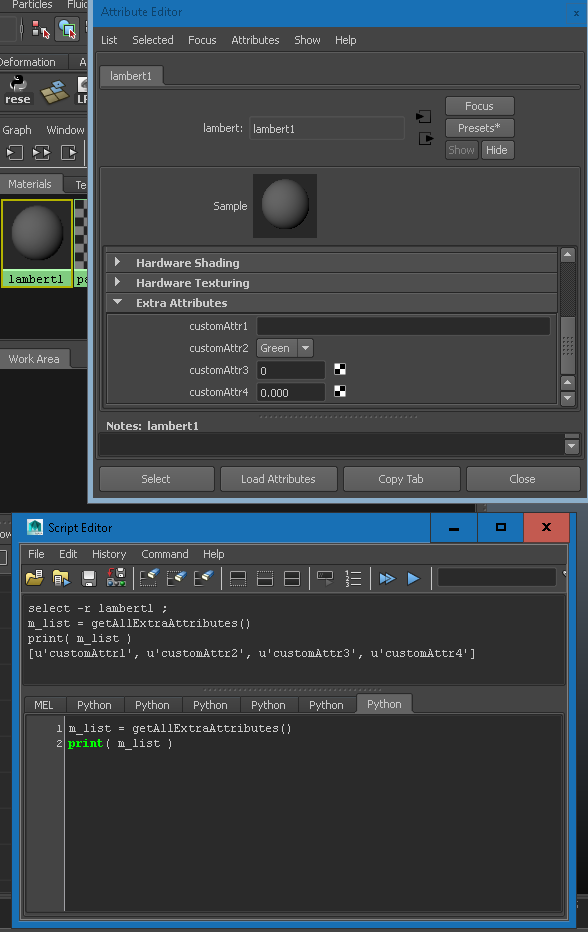#### Get all attributes from the kFileTexture node Maya Python API

```
import maya.OpenMaya as OpenMaya

m_iterator = OpenMaya.MItDependencyNodes( OpenMaya.MFn.kFileTexture )
m_nodeFn   = OpenMaya.MFnDependencyNode()

while not m_iterator.isDone():
m_object = m_iterator.thisNode()
m_nodeFn.setObject( m_object )
print( "  --- {0} --- ".format( m_nodeFn.name()) )
for i in range( m_nodeFn.attributeCount() ):
m_atrr   = m_nodeFn.attribute(i)
m_fnAttr = OpenMaya.MFnAttribute( m_atrr )
print( m_fnAttr.name() )
m_iterator.next()
```